# Partial Residuals and the termplot function

Earlier this year I taught an Intro to R Graphics workshop. While preparing for it I came across the termplot function. I had no idea it existed. Of course now that I know about it, I come across it fairly regularly. (That's the Baader-Meinhof Phenomenon for you.)

?termplot will tell you what it does: “Plots regression terms against their predictors, optionally with standard errors and partial residuals added.” If you read on, you'll see “Nothing sensible happens for interaction terms, and they may cause errors.” Duly noted.

Let's try this out and see what it does.

First we'll fit a model using the mtcars dataset that comes with R. Below mpg = Miles/(US) gallon, drat = Rear axle ratio, qsec = ¼ mile time, disp = Displacement (cu.in.), and wt = Weight (lb/1000).

lm.cars <- lm(mpg ~ wt + disp + drat + qsec, data=mtcars)
summary(lm.cars)

##
## Call:
## lm(formula = mpg ~ wt + disp + drat + qsec, data = mtcars)
##
## Residuals:
##    Min     1Q Median     3Q    Max
## -4.143 -1.933 -0.149  0.919  5.541
##
## Coefficients:
##             Estimate Std. Error t value Pr(>|t|)
## (Intercept)  9.03223    9.58829    0.94  0.35454
## wt          -4.86768    1.20872   -4.03  0.00041 ***
## disp         0.00522    0.01105    0.47  0.64021
## drat         1.87086    1.32462    1.41  0.16926
## qsec         1.05248    0.34778    3.03  0.00539 **
## ---
## Signif. codes:  0 '***' 0.001 '**' 0.01 '*' 0.05 '.' 0.1 ' ' 1
##
## Residual standard error: 2.6 on 27 degrees of freedom
## Multiple R-squared:  0.838,  Adjusted R-squared:  0.814
## F-statistic:   35 on 4 and 27 DF,  p-value: 2.55e-10

par(mfrow=c(2,2))
termplot(lm.cars)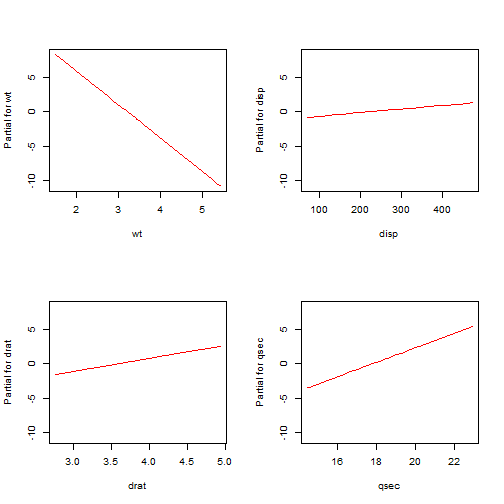Compare the coefficients in the summary output to the slopes of the lines in the graphs. See how the coefficients match the slopes of the lines? That's because they are the slopes of the lines. For example, wt has a coefficient of about -5. Likewise the termplot for that coefficient has a negative slope that appears to be about -5. Using these plots we can see at a glance the respective contributions of the predictors. wt and qsec seem to contribute to the model due to the steepness of their slopes, but disp and drat not so much. This matches the summary output that shows wt and qsec as being significant.

Now termplot also has an argument called partial.resid that will add partial residuals if set to TRUE. Let's try that out, with color set to “purple” since the default gray can be hard to see (at least to my eyes):

par(mfrow=c(2,2))
termplot(lm.cars, partial.resid=TRUE, col.res = "purple")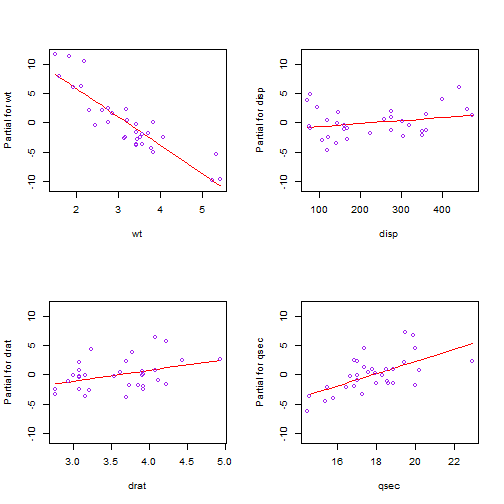How are these points generated? The x-axis is clear enough. That's just the predictor values. But the y-axis says “Partial for [variable name]”, i.e. partial residuals. What does that mean? Put as simply as possible, partial residuals are the sum of the residuals and predictor terms. Hopefully you know what residuals are. Those are just the difference between the observed and fitted response values. “Predictor terms” is a little trickier.

To understand them, it helps to know that

$y = \bar{y} + b_{1}(x_{1} – \bar{x}_{1}) + b_{2}(x_{2} – \bar{x}_{2}) + b_{3}(x_{3} – \bar{x}_{3}) + b_{4}(x_{4} – \bar{x}_{4}) + e$

is another way of writing

$y = b_{0} + b_{1}x_{1} + b_{2}x_{2}+ b_{3}x_{3}+ b_{4}x_{4} + e$

So the first equation above has $$\bar{y}$$ as the intercept and centered predictors. If we center the predictors and fit a model, we'll see that's precisely what we get:

# center the variables
mtcars <- transform(mtcars, wtC = wt-mean(wt), dispC = disp-mean(disp),
dratC=drat-mean(drat), qsecC=qsec-mean(qsec))
# now fit model using centered predictors
lm.terms.cars <- lm(mpg ~ wtC + dispC + dratC + qsecC, data=mtcars)
coef(lm.terms.cars)

## (Intercept)
##       20.09

mean(mtcars$mpg)  ##  20.09  Now if we take the centered predictor values and multiply them by their respective coefficients, we get what are referred to in R as terms. For example, the terms for the first record in the data set are as follows: # take all coefficients but the intercept... coef(lm.terms.cars)[-1]  ## wtC dispC dratC qsecC ## -4.867682 0.005222 1.870855 1.052478  # ...and first record of data... lm.terms.cars$model[1,-1]

##               wtC  dispC  dratC  qsecC
## Mazda RX4 -0.5972 -70.72 0.3034 -1.389

# ...and multiply:
coef(lm.terms.cars)[-1]*t(lm.terms.cars$model[1,-1])  ## Mazda RX4 ## wtC 2.9072 ## dispC -0.3693 ## dratC 0.5677 ## qsecC -1.4616  So for the first record, the term due to wt is 2.91, the term due to disp is -0.37, and so on. These are the predictor terms I mentioned earlier. These are what we add to the residuals to make partial residuals. You'll be happy to know we don't have to center predictors and fit a new model to extract terms. R can do that for us on the original model object when you specify type="terms" with the predict function, like so: # notice we're calling this on the original model object, lm.cars pterms <- predict(lm.cars, type="terms") pterms[1,] # compare to above; the same  ## wt disp drat qsec ## 2.9072 -0.3693 0.5677 -1.4616  To calculate the partial residuals ourselves and make our own partial residual plots, we can do this: # add residuals to each column of pterms (i.e., the terms for each predictor) partial.residuals <- apply(pterms,2,function(x)x+resid(lm.cars)) # create our own termplot of partial residuals par(mfrow=c(2,2)) # the model in lm.cars includes the response in first column, so we index with i + 1 for(i in 1:4){ plot(x=lm.cars$model[,(i+1)],y=partial.residuals[,i], col="purple", ylim=c(-10,10))
abline(lm(partial.residuals[,i] ~ lm.cars$model[,(i+1)]), col="red") }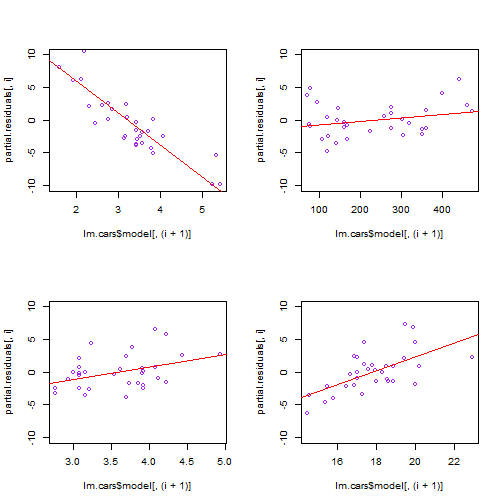So that's what termplot does for us: it takes the terms for each predictor, adds the residuals to the terms to create partial residuals, and then plots partial residuals versus their respective predictor, (if you specify partial.resid=TRUE). And of course it plots a fitted line, the result of regressing the predictor's partial residuals on itself. Recall ealier I mentioned the slope of the line in the termplot graphs is the coefficient in the summary output. We can indeed verify that: # coefficient for wt coef(lm.cars)  ## wt ## -4.868  coef(lm(partial.residuals[,1] ~ mtcars$wt))

## mtcars$wt ## -4.868  Ultimately what this whole partial residual/termplot thing is doing is splitting the response value into different parts: an overall mean, a term that is due to wt, a term that is due to disp, a term that is due to drat, and a term that is due to qsec. And you have the residual. So when we create the partial residual for wt, what we're doing is adding the wt term and the residual. This sum accounts for the part of the response not explained by the other terms. Again let's look at the overall mean, the terms for the first observation and its residual: # overall mean of response mean(mtcars$mpg)

##  20.09

# terms of first observation
pterms[1,]

##      wt    disp    drat    qsec
##  2.9072 -0.3693  0.5677 -1.4616

# the residual of the first observation
resid(lm.cars)

## Mazda RX4
##   -0.7346


If we add those up, we get the observed value

# add overall mean, terms and residual for first observation
mean(mtcars$mpg) + sum(pterms[1,]) + resid(lm.cars)  ## Mazda RX4 ## 21  # same as observed in data mtcars$mpg

##  21


So the wt term value of 2.9072 represents the part of the response that is not explained by the other terms.

Finally, we can add another argument to termplot, smooth=panel.smooth that will draw a smooth lowess line through the points. This can help us assess departures from linearity.

par(mfrow=c(2,2))
termplot(lm.cars, partial.resid=TRUE, col.res = "purple", smooth=panel.smooth)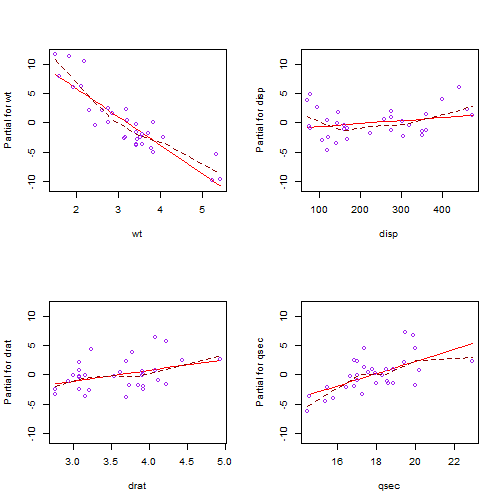Notice how the dashed line bends around the straight line for wt. Perhaps wt requires a quadratic term? Let's try that using the poly function, which creates orthogonal polynomials.

lm.cars2 <- lm(mpg ~ poly(wt,2) + disp + drat + qsec, data=mtcars)
par(mfrow=c(2,2))
termplot(lm.cars2, partial.resid=TRUE, col.res = "purple", smooth=panel.smooth)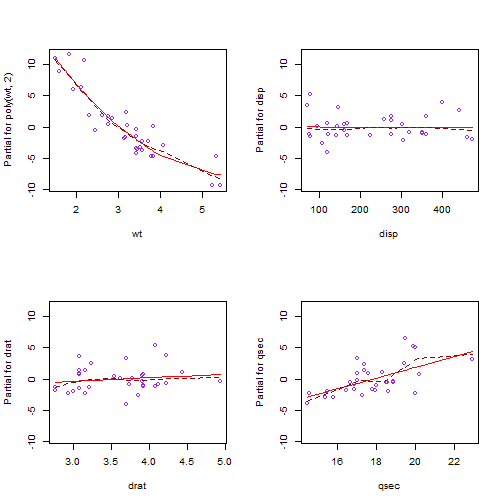We now see a better fit with the regressed line matching closely to the smooth line.

## 8 thoughts on “Partial Residuals and the termplot function”

1. An Appreciative Reader

Thanks for a delightful and very clear exposition re: termplot.
It answered all my questions, and provided some very nice examples.

FYI, found a small typo: please replace

“Preditor terms” is a little trickier.

with

“Predictor terms” is a little trickier.

Thanks. /AAR

2. Laura

Thanks for this post, very useful!
I am trying to do the same graphs for independent variables in my Linear Mixed Model. Could you possibly give me few suggestions? Thank you very much!

1. Clay Ford Post author

Hi Laura.

I don’t think this is something one routinely does for linear mixed-effect models due to the grouping structure and additional error terms. Let’s say you had a random intercept model and 20 groups. You would have 20 sets of within-group residuals, which I think would mean creating 20 different termplots. That sounds pretty horrible. Not saying it can’t be done, just saying I’ve never done it and don’t have any suggestions for you. Sorry.

Clay

3. Amrit

Why is the intercept not added to the partial residuals to make the y-axis more interpretable?
A

1. Clay Ford Post author

I’m not sure I understand your question. Do you mean why doesn’t the x-axis go all the way to 0 so we can see where the fitted line crosses the y-axis? If so, that’s because the partial residuals are for the predictor values, and the predictor values don’t come close to 0. I’m not sure how helpful it is to see the fitted line extrapolated to a predictor value set to 0, at least not for these car data.

4. NKSimons

Dear Clay Ford,

thanks for this post, it really helped a lot to see and work through the code to produce the termplot myself to understand what it shows.
However, when I applied this to my own data, I found that the slopes in the termplots were very different from the coefficients of my model. After trying to find out where my mistake was, I discovered that the slopes in the termplot do not reflect the coefficients. It matches pretty well in your example but this is just a coincidence because the variable wt as low values (i.e. up to 5 in comparison to disp which has values up to 400) and a high coefficient.
If you try the same procedure with e.g. hp instead of wt, the model coefficients and the slopes do not match (unfortunately, I cannot paste the plot here). What the termplot actually shows is the relative importance of a variable to the whole model. If we look at the model summary of a model with hp, we see that hp and disp have smaller coefficients than qsec but the slopes in the termplot are steeper. This is because if you multiply the mean of hp with its coefficient, it results in a larger negative value than when you multiply the mean of qsec with its coefficient (see below). Hence, hp has a greater influence on the predicted value than qsec, which is visible in the termplot but not in the coefficients.

I hope this helps other readers, which might stumble over the same problem with their own data.

Here is some code with hp instead of wt:

> summary(lm.cars)
Call: lm(formula = mpg ~ hp + disp + drat + qsec, data = mtcars)

Residuals:
Min 1Q Median 3Q Max
-4.9468 -1.8845 -0.5952 1.1165 6.6771

Coefficients:
Estimate Std. Error t value Pr(>|t|)
(Intercept) 24.241299 12.819746 1.891 0.0694 .
hp -0.036108 0.017464 -2.068 0.0484 *
disp -0.018879 0.009542 -1.978 0.0582 .
drat 2.555409 1.551713 1.647 0.1112
qsec -0.206682 0.467241 -0.442 0.6618

> mean(mtcars$hp)  146.6875 > mean(mtcars$qsec)
 17.84875

> mean(mtcars$hp)*coef(lm.cars) hp -5.296594 ## –> bigger effect even though the coefficient is smaller > mean(mtcars$qsec)*coef(lm.cars)
qsec
-3.68901

1. Clay Ford Post author

I think you missed a step along the way. When I try the same procedure with hp instead of wt, the model coefficients and the termplot slopes still match.

> # fit model with hp
> lm.cars <- lm(mpg ~ hp + disp + drat + qsec, data = mtcars)
> # coefficient of hp for model
> coef(lm.cars)
hp
-0.03610801
> # get terms
> pterms <- predict(lm.cars, type=”terms”)
> # calculate partial residuals
> partial.residuals <- apply(pterms,2,function(x)x+resid(lm.cars))
> # get “slope” of termplot
> # same as model coefficient
> lmout <- lm(partial.residuals[,1] ~ mtcars$hp) > coef(lmout) mtcars$hp
-0.03610801

This site uses Akismet to reduce spam. Learn how your comment data is processed.